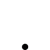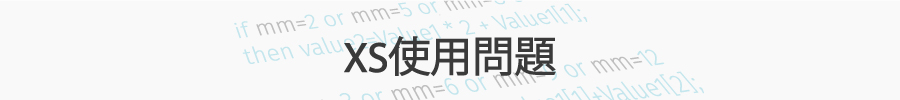# 想寫統計某段時間內分公司買進少於賣出的家數??

•   103
• 最後發表   topgolei  2018 一月 17
topgolei 發文於   2018/01/13

input:period(3);

variable:_count(0);

Value1=GetField("分公司買進家數","D");

value2=GetField("分公司賣出家數","D");

value4=value1-value2;

settotalbar(10);

for value3=0 to period

begin

if value4[value3]<0 then

_count=_count + 1;

end ;

if _count>=3 then ret=1;

outputfield(1,value1,"分公司買進家數1");

outputfield(2,value2,"分公司賣出家數1");

outputfield(3,value1,"分公司買進家數2");

outputfield(4,value2,"分公司賣出家數2");

outputfield(5,value1,"分公司買進家數3");

outputfield(6,value2,"分公司賣出家數3");

outputfield(7,_count,"次數");

outputfield(8,value3,"for次數");

XQ小幫手 發文於   2018/01/16

Q1：那是因為0也算是一期喔，所以0-3是4期沒錯。

Q2：這是因為你的count沒有歸0的問題

Q3：同Q2

```input:period(3);

variable:_count(0);

Value1=GetField("分公司買進家數","D");

value2=GetField("分公司賣出家數","D");

value4=value1-value2;

settotalbar(10);
_count=0;

for value3=0 to period-1

begin

if value4[value3]<0 then

_count=_count + 1;

end ;

if _count=3 then ret=1;

outputfield(1,value1,"分公司買進家數1");

outputfield(2,value2,"分公司賣出家數1");

outputfield(3,value1,"分公司買進家數2");

outputfield(4,value2,"分公司賣出家數2");

outputfield(5,value1,"分公司買進家數3");

outputfield(6,value2,"分公司賣出家數3");

outputfield(7,_count,"次數");

outputfield(8,value3,"for次數");```

topgolei 發文於   2018/01/16

`這個不是代表我宣告的變數 _count為0 的意思嗎?? 為何還要再多一行 _count=0;`

`因為我看程式交易的七堂課的投影片裡面寫 Variable:變數名稱(初始值);初始值是數值或布林值`

`所以我才沒寫_count=0; 這行`

XQ小幫手 發文於   2018/01/17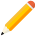發表新主題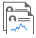我的發文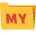我的收藏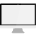分類

Close